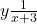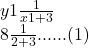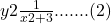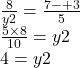Question

.y is inversely proportional to (x + 3) ?.
When x = 2, y = 8.
Find y when x = 7.
A 100
B. 4
C. 2.
D. 16
OA

1.B

Step-by-step explanation:

y = k/(x + 3)

Let x = 2

Let y = 8

k = ?

8 = k/(2 + 3)      Combine the terms on the right

8 = k/(5)            Multiply both sides by 5

8*5 = k

k = 40

Let x = 7

y = 40/(7 + 3)

y = 40/10

y = 4

2.DIVIDE BOTH THE EQUATION